# Graphing Functions

Graphing Functions

Do you know any interesting facts about graphing functions?

Here's one for you!

Did you know the concept of functions was introduced in the 17th century along with the development of calculus?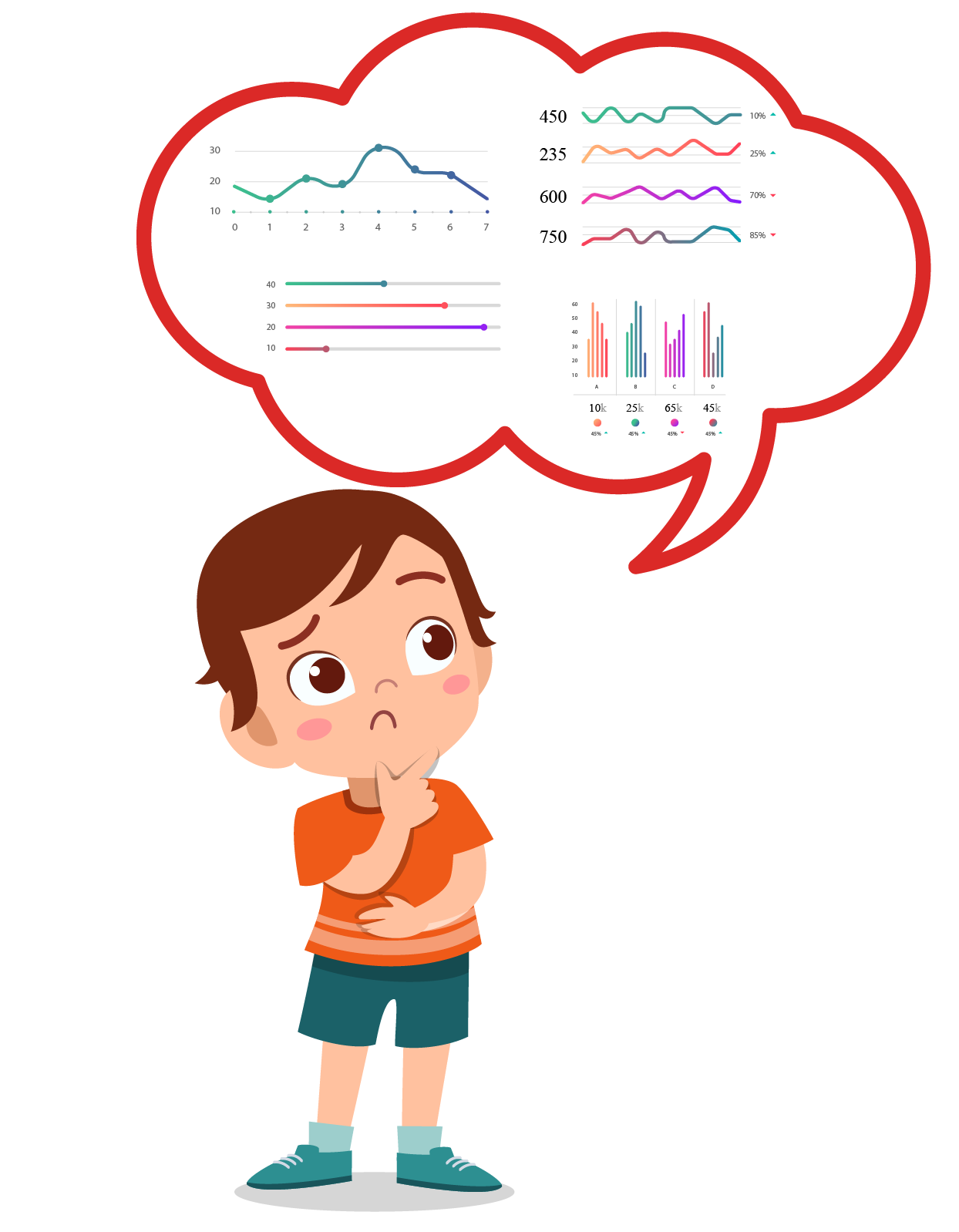In this mini-lesson, we will explore the world of graphing functions by learning about graphing functions rules, graphing functions calculator, graphing functions algebra 1, and algebra 2 graphing functions while discovering interesting facts around them.

## Lesson Plan

 1 How Do You Know If the Graph Is a Function? 2 Tips and Tricks 3 Solved Examples on Graphing Functions 4 Interactive Questions on Graphing Functions 5 Challenging Questions on Graphing Functions

## How Do You Know If the Graph Is a Function?

Before we go ahead, let us recall what we have learnt about functions

To know whether a graph is a function or not, we do a vertical line test.

For this test, a vertical line is drawn across the graph, however, it must cut the graph only once.

If the vertical line drawn cuts the graph at more than one place, then it shows that for the same value of input, there is more than one output which indicates the input and output do not have a well-defined relation.

## What Is the Function of this Graph Calculator?

The graphing functions calculator is used to derive a graph from the given function.

It follows all the graphing function rules.

Have a look at the below given graphing functions calculator.

Let's say we have a function $$f(x)$$.

Let's see what happens when the graphing functions rules are applied:

Transformation Change in graph
$$f(x) + c$$ It shifts the graph of the function $$c$$ units upward.
$$f(x) - c$$ It shifts the graph of the function $$c$$ units downward.
$$f(x + c)$$ It shifts the graph of the function $$c$$ units to the left.
$$f(x - c)$$ It shifts the graph of the function $$c$$ units to the right.
$$- f(x)$$ It reflects the graph of the function in the $$x$$ axis (upside down).
$$f(-x)$$ It reflects the graph of the function in the $$y$$ axis (i.e., the left and right sides are swapped).Tips and Tricks

## Types of Functions

The various types of functions are:

1. Identity Function
2. Constant Function
3. Polynomial Function
5. Cubic Function
6. Rational Function
7. Modulus Function

From the above functions, identity functions, constant functions, and polynomial functions come under graphing functions algebra 1 while quadratic functions, cubic functions, rational functions, and modulus functions come under algebra 2 graphing functions.

### Identity Function

Identity function is the type of function which gives the same input as the output.

It is expressed as,

$$f(x) = x$$, where $$x \in \mathbb{R}$$

For example, $$f(3) = 3$$ is an identity function.

### Constant Function

Constant function is the type of function which gives the same value of output for any given input.

It is expressed as,

$$f(x) = c$$, where $$c$$ is a constant

For example, $$f(x) = 2$$ is an identity function.

### Polynomial Function

Polynomial function is a type of function which can be expressed as a polynomial.

It is expressed as,

$$f(x) = a_{n}x^{n} + a_{n-1}x^{n-1} +....... + a_{0}x^{0}$$

For example, $$f(x) = 2x+5$$ is a polynomial function.

Quadratic function is a type of function which has the highest power 2 in the polynomial function.

It is expressed as,

$$f(x) = ax^{2} + bx +c$$

For example, $$f(x) = x^2 + 1$$ is a quadratic function.

### Cubic Function

Cubic function is a type of function which has the highest power 3 in the polynomial function.

It is expressed as,

$$f(x) = a_{1}x^{3} + a_{2}x^{2} + a_{3}x + a_{4}$$

For example, $$f(x) = x^3 + 4$$ is a cubic function.

### Rational Function

Rational function is a type of function which is derived from the ratio of two polynomial functions.

It is expressed as,

$$f(x) = \frac{P(x)}{Q(x)}$$, such that P and Q are polynomial functions of $$x$$ and $$Q(x)\neq0$$

For example, $$f(x) = \frac{x^2 + 2x + 1}{x^2 - 4}$$ is a rational function.

### Modulus Function

Modulus function is a type of function which gives the absolute value of a number by giving its magnitude.

It is expressed as,

$$f(x) = |x|$$

It can further be defined as:

$$\begin{equation*} f(x) = \begin{cases} x & x \geq 0\\ -x & x < 0\\ \end{cases} \end{equation*}$$

## Identify Functions Using Graphs

To identify functions, we normally use two tests:

1. Vertical line test
2. Horizontal line test

Let's understand each one of them.

1. Vertical line test

The vertical line test is used to check whether the given graph is a function or not.

For this test, a vertical line is drawn across the graph, however, it must cut the graph only once.

If the vertical line drawn cuts the graph at more than one place, then it shows that for the same value of input, there is more than one output which indicates that the input and output do not have a well-defined relation.

1. Horizontal line test

The horizontal line test is used to check whether the given graph represents a one-to-one function or not.

For this test, a horizontal line is drawn across the graph, however, it must cut the graph only once which is an indicator that for every input there is one output.

If the horizontal line drawn cuts the graph at more than one place, then it shows that the given graph is not a one-to-one function.

Look at the graphs shown below to understand the above concept in more detail.

Name of function Function Graph
Identity Function $$f(x) = x$$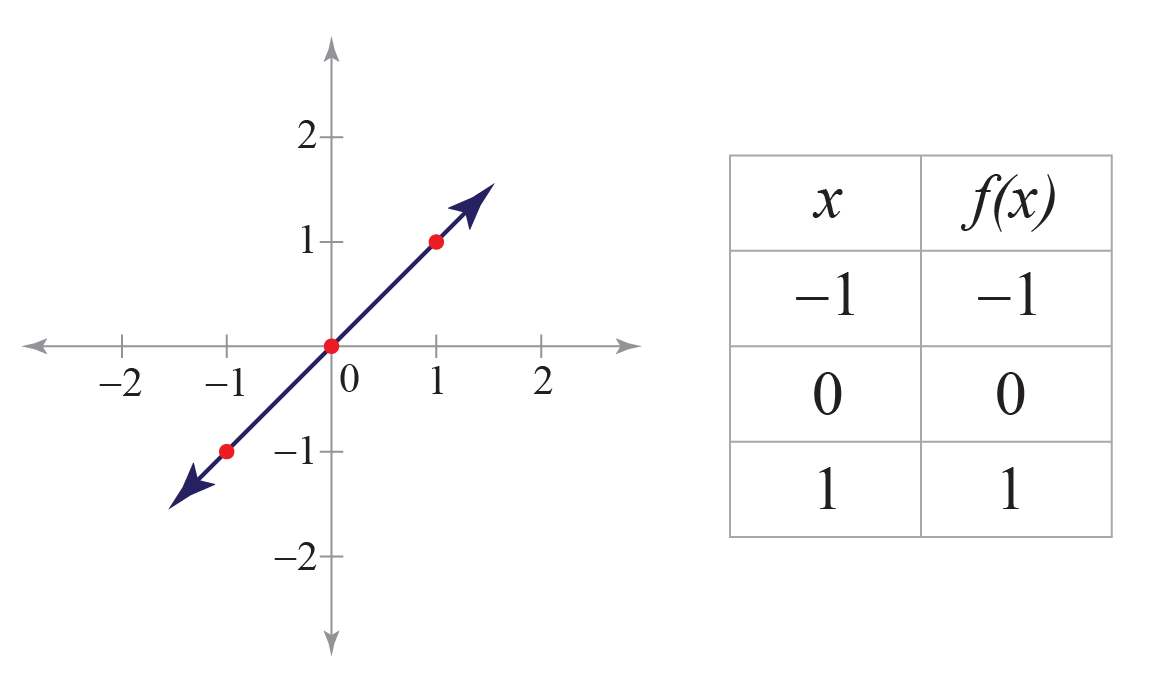Constant Function $$f(x) = c$$, where $$c$$ is constant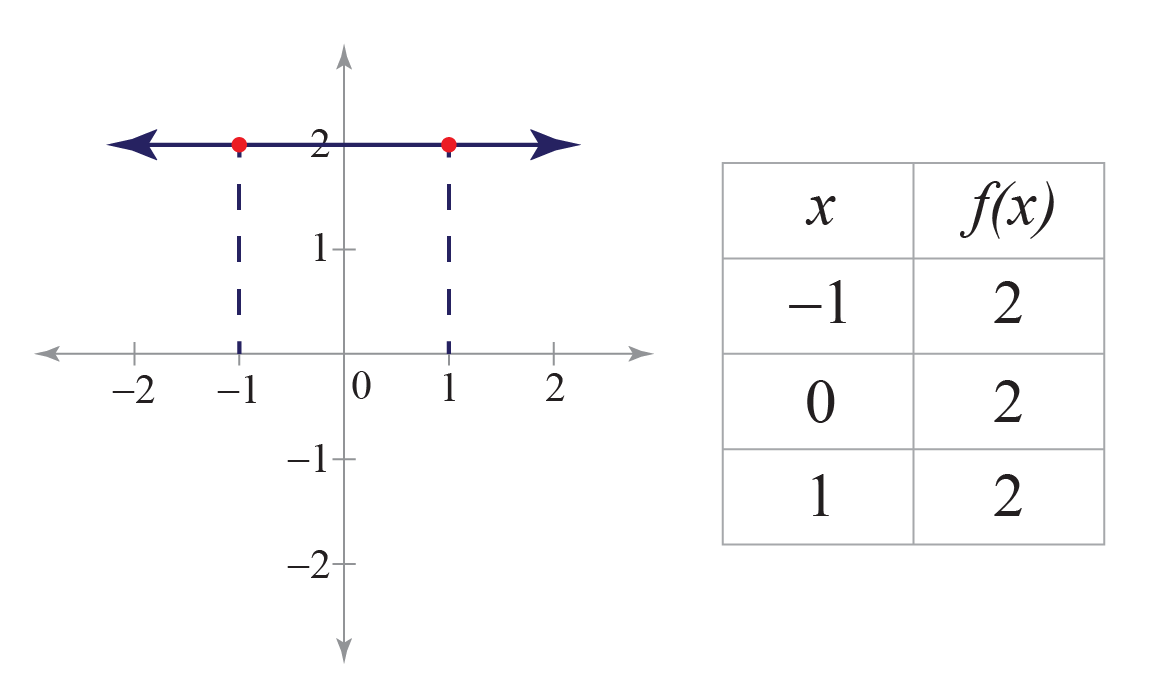Quadratic Function $$f(x) = x^{2}$$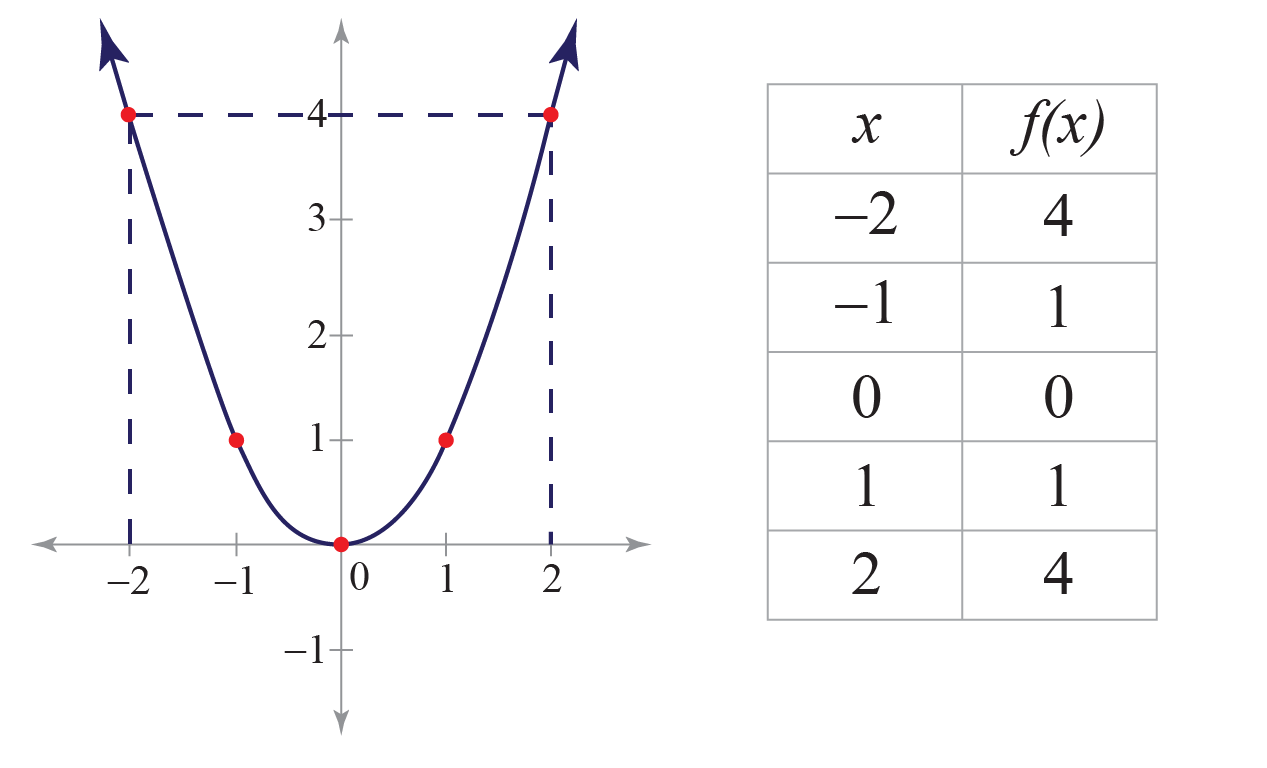Cubic Function $$f(x) = x^{3}$$Rational Function $$f(x) = \frac{1}{x}$$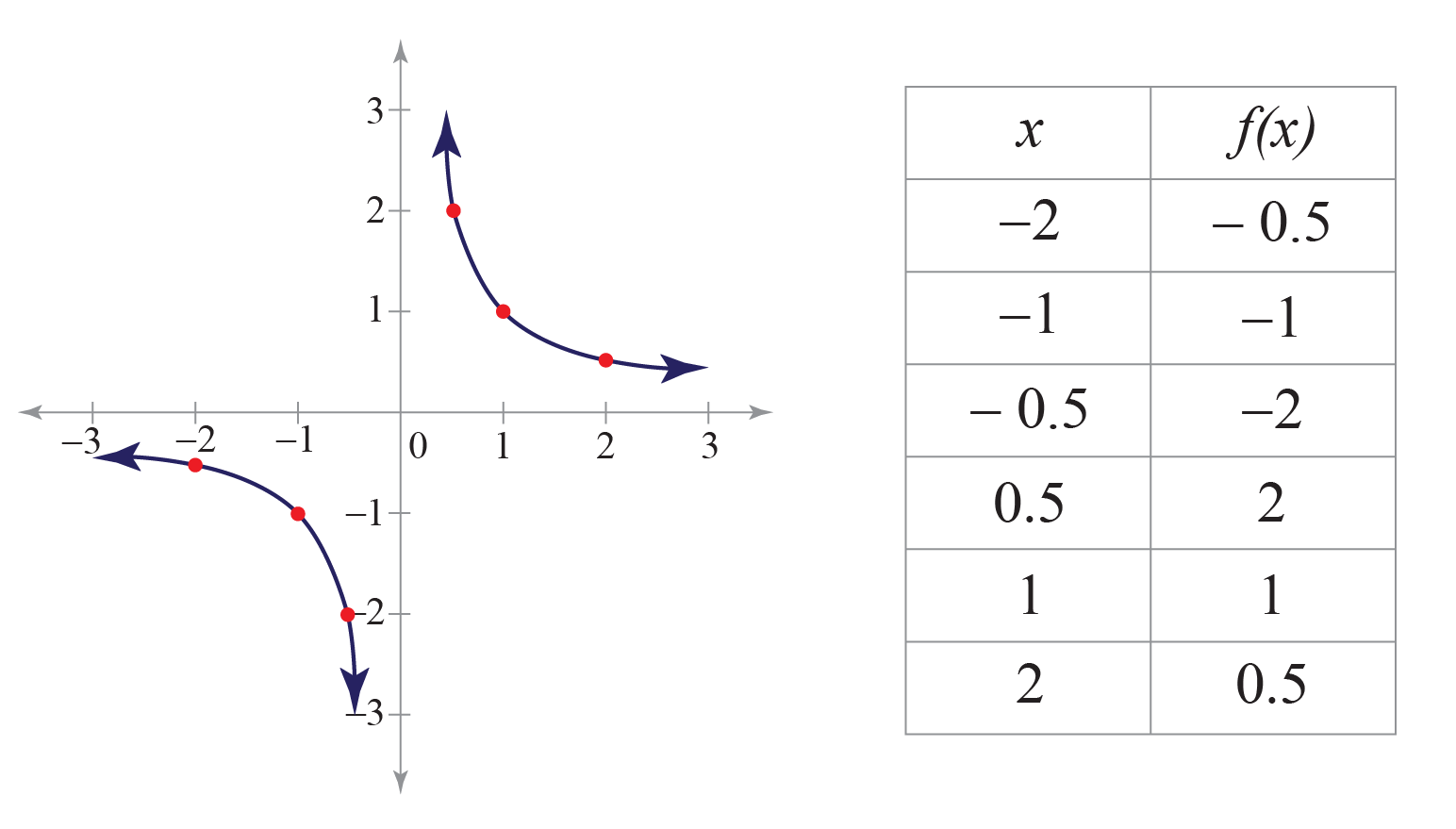Modulus Function $$f(x) = |x|$$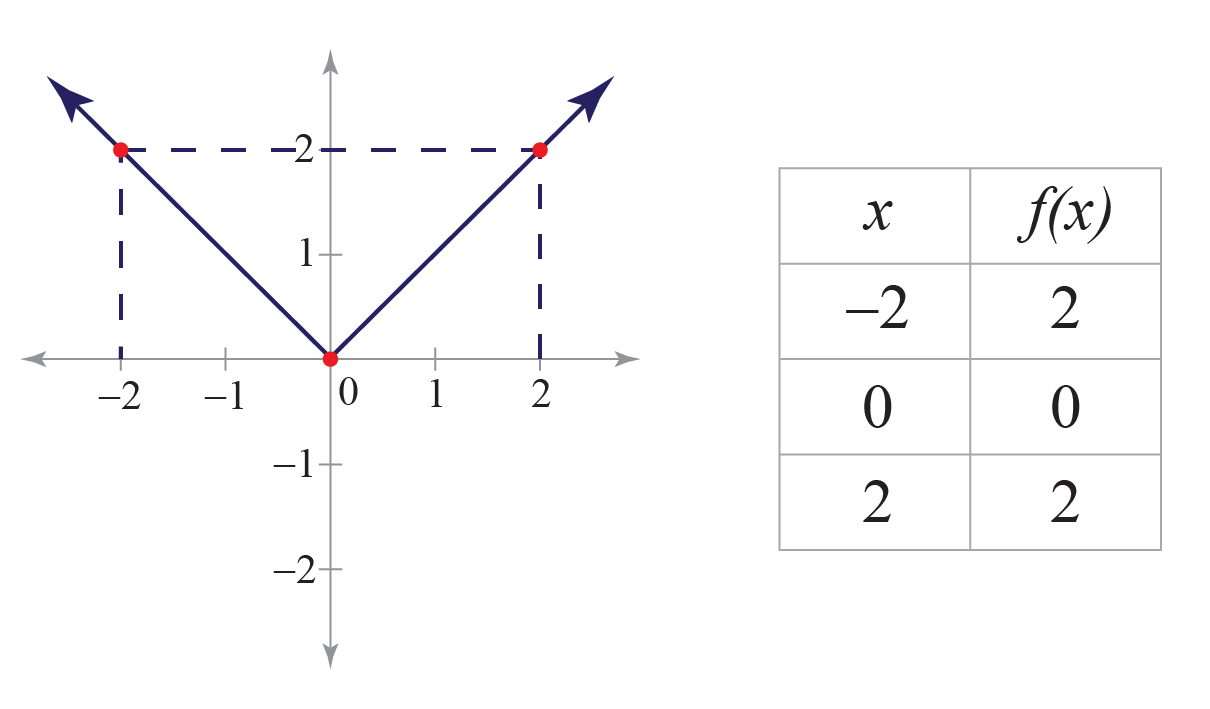## Solved Examples

 Example 1

How can Joey justify whether any given graph is a function or not?

Solution

Joey can use the vertical line test to justify whether any given graph is a function or not.

For this test, he will have to draw a vertical line across the graph.

If the line cuts the graph only once, then it is a function, else it is not a function.

 $$\therefore$$ He will use the vertical line test.
 Example 2

Isabella has to plot the function $$f(x) = x^2 + 3$$ and determine whether it is a one-to-one function. How should she proceed?

Solution

Isabella will assume $$y = f(x)$$.

To plot the graph, she will find the value of $$y$$ for various values of $$x$$.

Values of $$x$$ Values of $$y$$
-1 4
0 3
1 4

She can draw the graph as shown below.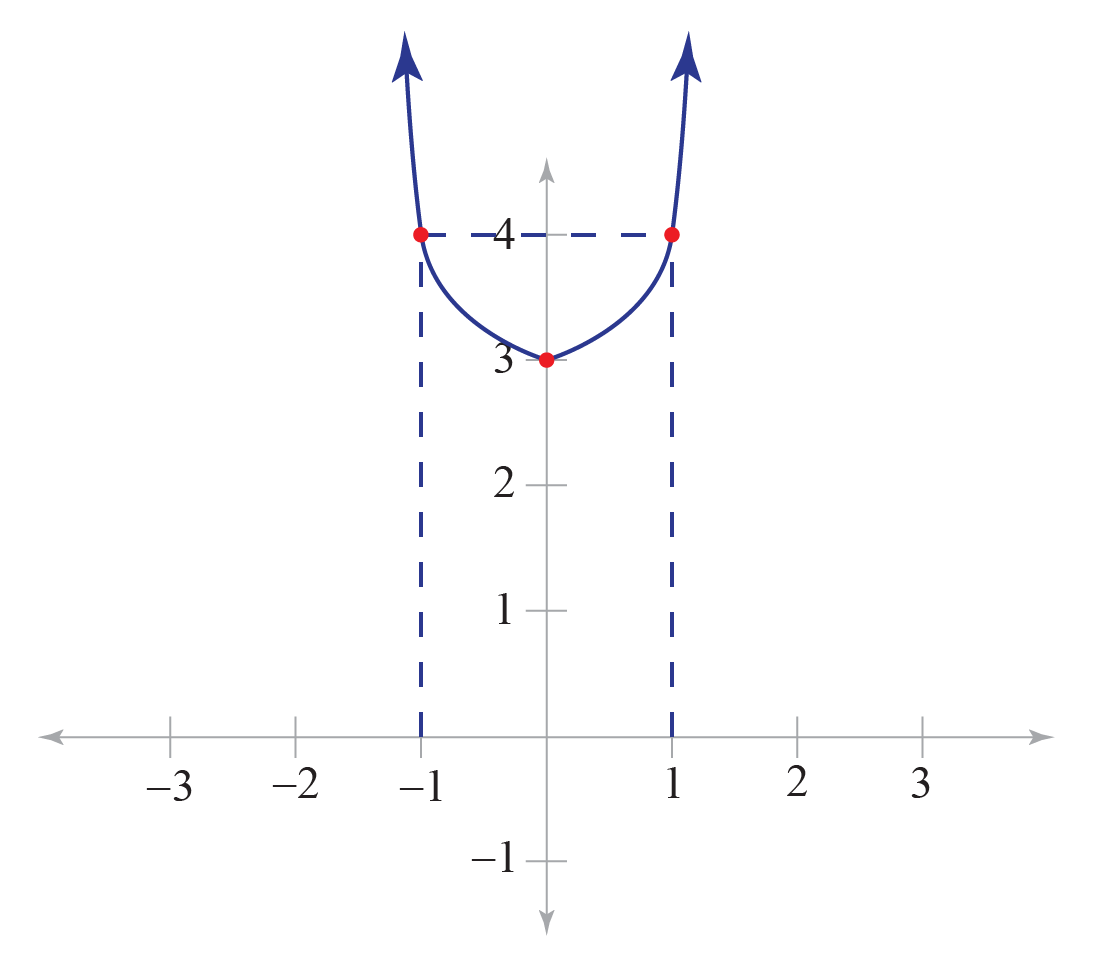To prove whether it is a one-to-one function or not, Isabella will do the horizontal line test.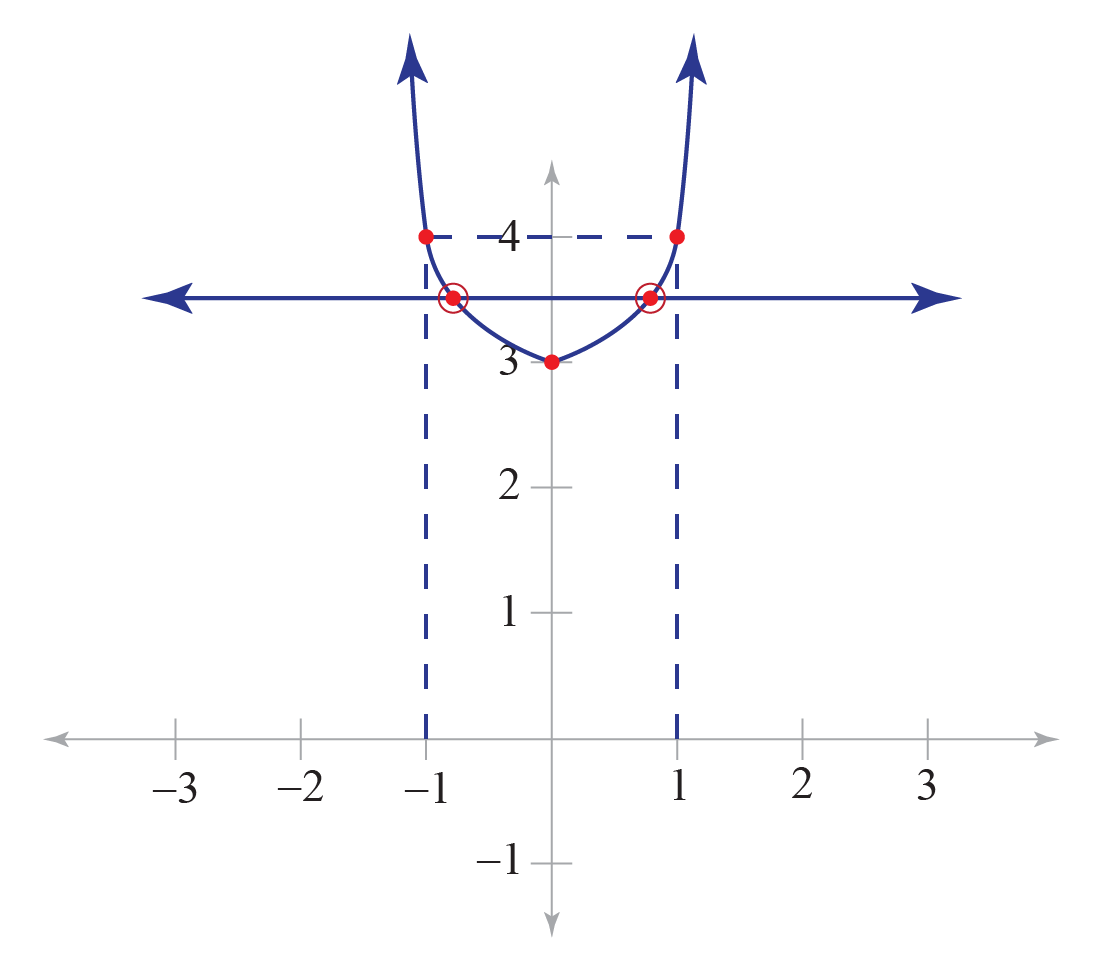The circled points indicate the number of intersections which are two in number.

Hence, she will conclude that the given function is not a one-to-one function as the line cuts at two points.

 $$\therefore$$ The given function is not a one-to-one function.
 Example 3

Can Anthony prove $$f(2x) \neq 2f(x)$$ if $$f(x) = x^3 + 2$$?

Solution:

Anthony knows $$f(x) = x^3 + 2$$.

To find $$f(2x)$$, he will substitute $$x$$ by $$2x$$.

He will get, $$f(2x) = (2x)^{3} + 2 = 8x^{3} + 2$$

To find $$2f(x)$$, he will multiply $$f(x)$$ by 2.

He will get, $$2f(x) = 2\times(x^{3} + 2) = 2x^{3} + 4$$

Thus, $$8x^{3} + 2 \neq 2x^{3} + 4$$

 $$\therefore$$ $$f(2x) \neq 2f(x)$$

## Interactive Questions

Here are a few activities for you to practice.Challenging Questions

How will Charlie plot the below given functions and check whether they are one-to-one functions or not?

1. $$f(x) = 4x^{4} + 2x + 1$$
2. $$f(x) = |x| + 2x^2 + 5$$

## Let's Summarize

The mini-lesson targeted the fascinating concept of graphing functions. The math journey around graphing functions starts with what a student already knows, and goes on to creatively crafting a fresh concept in the young minds. Done in a way that not only it is relatable and easy to grasp, but also will stay with them forever. Here lies the magic with Cuemath.

At Cuemath, our team of math experts is dedicated to making learning fun for our favorite readers, the students!

Through an interactive and engaging learning-teaching-learning approach, the teachers explore all angles of a topic.

Be it worksheets, online classes, doubt sessions, or any other form of relation, it’s the logical thinking and smart learning approach that we, at Cuemath, believe in.

## 1. What are the steps to graphing a linear function?

The steps to graph a linear function are given as follows:

• Make sure the linear function is of the form $$y = mx+b$$.
• Now, $$b$$ is plotted on $$y$$ axis.
• $$m$$ is converted into a fraction.
• Now the line is extended from $$b$$ using the slope.
• The line can further be extended using $$m$$ as a guiding factor.

## 2.  How do you use a graph to solve an equation?

An equation can be solved by checking where the curves intersect.

## 3. How do you sketch a graph of an equation?

To sketch a graph of an equation, do the following:

• Plot various points for the equation.
• Join the points and form the curve.
The curve so obtained is a graph of the given equation.
More Important Topics
Numbers
Algebra
Geometry
Measurement
Money
Data
Trigonometry
Calculus
More Important Topics
Numbers
Algebra
Geometry
Measurement
Money
Data
Trigonometry
Calculus
Learn from the best math teachers and top your exams

• Live one on one classroom and doubt clearing
• Practice worksheets in and after class for conceptual clarity
• Personalized curriculum to keep up with school